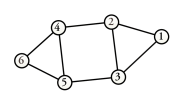# 1.3 Undirected Graph

If the edges of $G$ are unordered pairs, $G$ is a undirected graph. In an undirected graph, $(v,w)=(w,v)$. If edge $e$ of an undirected graph is represented by $(v,w)$, then $v$ is adjacent to $w$ and $w$ is adjacent to $v$. The terminology “undirected graph” is somewhat cumbersome. In this document, undirected graphs are often referred to as just “graphs”.

Figure 2 depicts an undirected graph with six vertices and eight edges. The fact that the graph is undirected is indicated by the absence of directionality arrows.

Figure 2: Example of an Undirected Graph